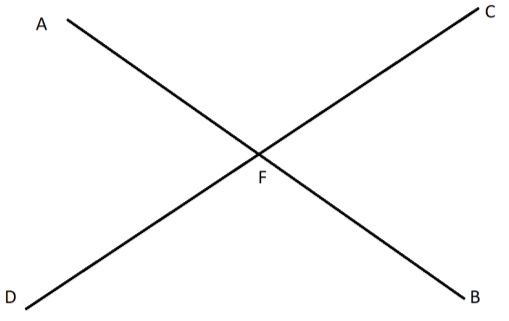Courses
Courses for Kids
Free study material
Free LIVE classes
More# Lines AB and CD intersect at a point F. $\angle AFC$ is ${m^ \circ }$, what are the measures of the other angles in the terms of ${m^ \circ }$.

Last updated date: 26th Mar 2023
Total views: 207.3k
Views today: 2.83kVerified
207.3k+ views
Hint: We first draw two intersecting lines and we name them as AB and CD. We name the point of intersection as F. We already know that angles of the same side of a line at a point are 180 degrees. We also know that the vertically opposite angles are equal. From this information we can get the measures of the angles.

Complete step-by-step solution:$\angle AFC$= ${m^ \circ }$.
As AB is a straight line and$\angle AFC$, $\angle CFB$ are angles of the same side of the line.
$\angle AFC + \angle CFB = {180^ \circ } \\ \Rightarrow {m^ \circ } + \angle CFB = {180^ \circ } \\ \Rightarrow \angle CFB = {180^ \circ } - {m^ \circ }$
( $\angle AFC$,$\angle BFD$ ) and ($\angle AFD$,$\angle CFB$) are vertically alternate interior and exterior opposite angles
$\angle AFC = \angle BFD = {m^ \circ } \\ \angle AFD = \angle CFB = {180^ \circ } - {m^ \circ }$
Hence, we get all the angles in form of $m^\circ$ such as:
$\angle AFC = {m^ \circ } \\ \angle CFB = {180^ \circ } - {m^ \circ } \\ \angle BFD = {m^ \circ } \\ \angle AFD = {180^ \circ } - {m^ \circ } \\$

Note: As the line is straight , so its angle is 180 degrees . The sum of angles on the same side of line at a point is 180 degrees or the angles are supplementary . The sum of angle around the point is 360 degrees. The alternate interior and exterior angles are equal. Co-interior angles add up to 180 degrees.